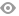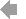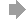﻿ Page 10 - 新思维数学 宣传册
Basic HTML VersionView Full Version
Page 10 - 新思维数学 宣传册
P. 10

``````新思维数学 MATHEMATICS

课程体系                          共 148 个单元，覆盖数、代数、几何与测量、统计与概率四大方向

Stage             Number                   Geometry and measure        Statistics and probability
1 Numbers to 10   3 Fractions  2 Geometry   4 Measures   6 Position  7 Statistics
5 Working with numbers to 10   8 Time           10 Geometry (2)  14 Statistics (2)
1
9 Numbers to 20   11 Fractions (2)  12 Measures (2)   15 Time (2)
13 Working with numbers to 20  16 Position, direction and patterns
1 Numbers to 100               2 Geometry      3 Measures     4 Statistics
5 Working with numbers to 100  7 Time          11 Geometry (2)  9 Statistics (2)
2
6 Money     8 Numbers to 100 (2)  12 Telling the time   13 Measures (2)  14 Pattern and probability
10 Calculating                 15 Symmetry, position and movement
1 Numbers to 1000              4 3D shapes                    2 Statistics: tally charts and
3 Addition, subtraction and money  6 Measurement, area and perimeter  frequency tables
5 Multiplication and division  8 Time                         10 Graphs
3    7 Fractions of shapes          13 Measure                     16 Chance
9 More addition and subtraction  14 Time (2)
11 More multiplication and division  15 Angles and movement
12 More fractions              17 Pattern and symmetry
1 Numbers and the number system  2 Time and timetables        4 Probability
3 Addition and subtraction of whole   6 2D shapes             10 Collecting and recording data
numbers                      8 Angles                       16 Data display and interpretation
5 Multiplication, multiples and factors  12 Investigating 3D shapes and nets
7 Fractions                    14 Area and perimeter
4
9 Comparing, rounding and dividing  18 Position, direction and movement
11 Fractions and percentages
15 Special numbers
17 Multiplication and division
1 The number system            2 2D shape and pattern         4 Averages
3 Numbers and sequences        6 3D shapes                    8 Probability
5 Addition and subtraction     10 Angles                      12 Data
7 Fractions, decimals and percentages  14 Area and perimeter
5    9 Addition and subtraction of fractions  16 Time
11 Multiplication and division
18 Position and direction
13 Ratio and proportion
15 Multiplying and dividing fractions and
decimals
17 Number and the laws of arithmetic
1 The number system            5 2D shapes                    3 Averages
2 Numbers and sequences        7 Exploring measures           9 Probability
4 Addition and subtraction (1)  11 3D shapes                  15 Data
6 Fractions and percentages    13 Angles
6    8 Addition and subtraction (2)  17 Transformations
10 Multiplication and division (1)
12 Ratio and proportion
14 Multiplication and division (2)
16 The laws of arithmetic
Stage           Number              Geometry and measure  Statistics and probability  Algebra
1 Integers  3 Place value and rounding 5 Angles and constructions  6 Collecting data  2 Expressions, formulae
4 Decimals    7 Fractions    8 Shapes and symmetry  11 Graphs             and equations
7
10 Percentages               14 Position and transformation 13 Probability  9 Sequences and functions
12 Ratio and proportion      15 Shapes, area and volume  16 Interpreting results  11 Graphs
1 Integers  3 Place value and rounding 5 Angles and constructions  6 Collecting data  2 Expressions, formulae
4 Decimals    7 Fractions    8 Shapes and symmetry  11 Graphs   13 Probability  and equations
8
10 Percentages               14 Position and transformation 16 Interpreting and   9 Sequences and functions
12 Ratio and proportion      15 Distance, area and volume  discussing results  11 Graphs
1 Number and calculation     5 Angles               6 Statistical investigations  2 Expressions and
3 Decimals, percentages and rounding 7 Shapes and measurements  12 Probability  formulae
8 Fractions                  13 Position and transformation 15 Interpreting and   4 Equations and
9
11 Ratio and proportion      14 Volume, surface area and   discussing results  inequalities
symmetry                                  9 Sequences and functions
10 Graphs
10``````5   6   7   8   9   10   11   12